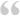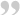Originally Said By: Jason ByrnesThe widths that the full size images and thumbnails are created at are set in the admin/common/properties.php file.

There are two arrays created, one for proportional images (mostly used by the large images):
php:
``````\$GLOBALS['resize_proportional'] = array(
array('w' => 1024, 'h' => 768, 'watermark' => true),
array('w' => 782, 'h' => 481, 'watermark' => true),
array('w' => 163, 'h' => 92)
);``````

another for cropped images (mostly used by the thumbnails):
php:
``````\$GLOBALS['resize_cropped'] = array(
array('w' => 62, 'h' => 62),
array('w' => 132, 'h' => 140),
array('w' => 102, 'h' => 102),
array('w' => 42, 'h' => 42),
array('w' => 782, 'h' => 481)
);``````

when you process the images to upload them, they are crated at the sizes in both arrays.

So to add 680 x 420 images for both thumb and large image, you would need to modify both arrays:
php:
``````\$GLOBALS['resize_proportional'] = array(
array('w' => 1024, 'h' => 768, 'watermark' => true),
array('w' => 782, 'h' => 481, 'watermark' => true),
array('w' => 163, 'h' => 92),
array('w' => 680, 'h' => 420, 'watermark' => true)
);
\$GLOBALS['resize_cropped'] = array(
array('w' => 62, 'h' => 62),
array('w' => 132, 'h' => 140),
array('w' => 102, 'h' => 102),
array('w' => 42, 'h' => 42),
array('w' => 782, 'h' => 481),
array('w' => 680, 'h' => 420)
);``````

Then, in the index.php file in the gallery directory you are using. On about line 14, there should be some code like this: &thumb=62c&full=782p.

"&thumb=62c" is telling it to use "array('w' => 62, 'h' => 62)," from the resize_cropped array for the thumbnail. "&full=782p" is telling it to use "array('w' => 782, 'h' => 481, 'watermark' => true)," from the resize_proportional array for the large images. to change that so the large image uses the 680 x 420 image we added:
&thumb=62c&full=680p.

the following how to has instructions for changing the number of images that are displayed in the ribbon:
powergallery_custom_gallery_ht.pdfit really looks simple but it does not work for me; i would like to make the thumbs bigger but after many trials i was not succesfull. I changed de 62 in 92, uploaded the images again and also changed the indec.php file but there is no change.
Someone any idea what i do wrong?

thanks,

PeterL